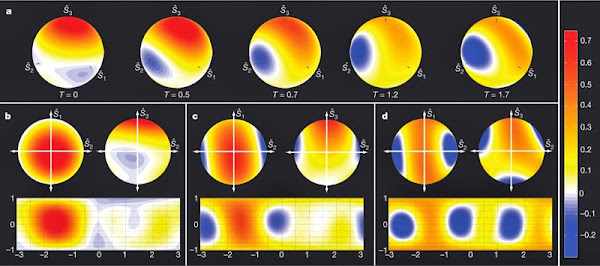### Triphoton Wigner quasi-probability distributions on the Poincaré sphereQuantum mechanics places a fundamental limit on the accuracy of measurements. In most circumstances, the measurement uncertainty is distributed equally between pairs of complementary properties; this leads to the 'standard quantumlimit' for measurement resolution. Using a technique known as 'squeezing', it is possible to reduce the uncertainty of one desired property below the standard quantumlimit at the expense of increasing that of the complementary one. Squeezing is already being used to enhance the sensitivity of gravity-wave detectors and may play a critical role in other high precision applications, such as atomic clocks and optical communications. Spin squeezing (the squeezing of angular momentum variables) is a powerful tool, particularly in the context of quantum light–matter interfaces. Although impressive gains in squeezing have beenmade, optical spin-squeezed systems are stillmany orders of magnitude away from the maximum possible squeezing, known as the Heisenberg uncertainty limit. Here we demonstrate how an optical system can be squeezed essentially all the way to this fundamental bound. We construct spin-squeezed states by overlapping three indistinguishable photons in an optical fibre and manipulating their polarization (spin), resulting in the formation of a squeezed composite particle known as a 'triphoton'. The symmetry properties of polarization imply that the measured triphoton states can be most naturally represented by quasi-probability distributions on the surface of a sphere. In this work we show that the spherical topology of polarization imposes a limit on how much squeezing can occur, leading to the quasi-probability distributions wrapping around the sphere — a phenomenon we term 'oversqueezing'. Our observations of spin-squeezing in the few-photon regime could lead to new quantum resources for enhanced measurement, lithography and information processing that can be precisely engineered photon-by-photon.
In optics, the Poincaré sphere is a graphical tool for visualizing different types of polarized light, and was introduced by Henri Poincaré in Theorie mathematique de la lumiere (archive.org).
Read also: spie.org | buffalo.edu | ipr.res.in